# On label dependence and loss minimization in multi-label classification

## Abstract

Most of the multi-label classification (MLC) methods proposed in recent years intended to exploit, in one way or the other, dependencies between the class labels. Comparing to simple binary relevance learning as a baseline, any gain in performance is normally explained by the fact that this method is ignoring such dependencies. Without questioning the correctness of such studies, one has to admit that a blanket explanation of that kind is hiding many subtle details, and indeed, the underlying mechanisms and true reasons for the improvements reported in experimental studies are rarely laid bare. Rather than proposing yet another MLC algorithm, the aim of this paper is to elaborate more closely on the idea of exploiting label dependence, thereby contributing to a better understanding of MLC. Adopting a statistical perspective, we claim that two types of label dependence should be distinguished, namely conditional and marginal dependence. Subsequently, we present three scenarios in which the exploitation of one of these types of dependence may boost the predictive performance of a classifier. In this regard, a close connection with loss minimization is established, showing that the benefit of exploiting label dependence does also depend on the type of loss to be minimized. Concrete theoretical results are presented for two representative loss functions, namely the Hamming loss and the subset 0/1 loss. In addition, we give an overview of state-of-the-art decomposition algorithms for MLC and we try to reveal the reasons for their effectiveness. Our conclusions are supported by carefully designed experiments on synthetic and benchmark data.

## Introduction

In contrast to conventional (single-label) classification, the setting of multi-label classification (MLC) allows an instance to belong to several classes simultaneously. At first sight, MLC problems can be solved in a quite straightforward way, namely through decomposition into several binary classification problems; one binary classifier is trained for each label and used to predict whether, for a given query instance, this label is present (relevant) or not. This approach is known as binary relevance (BR) learning.

However, BR has been criticized for ignoring important information hidden in the label space, namely information about the interdependencies between the labels. Since the presence or absence of the different class labels has to be predicted simultaneously, it is arguably important to exploit any such dependencies.

In current research on multi-label classification, it seems to be an opinio communis that optimal predictive performance can only be achieved by methods that explicitly account for possible dependencies between class labels. Indeed, there is an increasing number of papers providing evidence for this conjecture, mostly by virtue of empirical studies. Often, a new approach to exploiting label dependence is proposed, and the corresponding method is shown to outperform others in terms of different loss functions. Without questioning the potential benefits of exploiting label dependencies in general, we argue that studies of this kind do often fall short of deepening the understanding of the MLC problem. There are several reasons for this, notably the following:

• The notion of label dependence or “label correlation” is often used in a purely intuitive manner without giving a precise formal definition. Likewise, MLC methods are often ad-hoc extensions of existing methods for multi-class classification.

• Many studies report improvements on average, but without carefully investigating the conditions under which label dependencies are useful and when they are perhaps less important. Apart from properties of the data and the learner, for example, it is plausible that the type of performance measure is important in this regard.

• The reasons for improvements are often not carefully distinguished. As the performance of a method depends on many factors, which are hard to isolate, it is not always clear that the improvements can be fully credited to the consideration of label dependence.

• Moreover, a multitude of loss functions can be considered in MLC, and indeed, a large number of losses has already been proposed and is commonly applied as performance metrics in experimental studies. However, even though these loss functions are of a quite different nature, a concrete connection between the type of multi-label classifier used and the loss to be minimized is rarely established, implicitly giving the misleading impression that the same method can be optimal for different loss functions.

The aim of this paper is to elaborate on the issue of label dependence in more detail, thereby helping to gain a better understanding of the mechanisms behind MLC algorithms in general. Subsequent to a formal problem description in Sect. 2, we will propose a distinction between two different types of label dependence in MLC (Sect. 3). These two types will be referred to as conditional and marginal (unconditional) label dependence, respectively. While the latter captures dependencies between labels conditional to a specific instance, the former is a global type of dependence, independent of any concrete observation. In Sect. 4, we distinguish three different (though not necessarily disjoint) views on MLC. Roughly speaking, an MLC problem can either be seen as a set of interrelated binary classification problems or as a single multivariate prediction problem. Our discussion of this point will reveal a close interplay between label dependence and loss minimization. Theoretical results making this interplay more concrete are given in Sect. 5, where we analyze two specific but representative loss functions, namely the Hamming loss and the subset 0/1 loss. Furthermore, in Sect. 6, a selection of state-of-the-art MLC algorithms is revisited in light of exploiting label dependence and minimizing different losses. Using both synthetic and benchmark data, Sect. 7 presents several experimental results on carefully selected case studies, confirming the conclusions that were drawn earlier on the basis of theoretical considerations. We end with a final discussion about facts, pitfalls and open challenges on exploiting label dependencies in MLC problems. Let us remark that this paper combines material that we have recently published in three other papers (Dembczyński et al. 2010a; Dembczyński et al. 2010b; Dembczyński et al. 2010c). However, this paper discusses in more detail the distinction between marginal and conditional dependence and introduces the three different views on MLC. The risk minimizers for multi-label loss functions have been firstly discussed in Dembczyński et al. (2010a). The theoretical analysis of the two loss functions, the Hamming and the subset 0/1 loss, comes from Dembczyński et al. (2010c), however, the formal proofs of the theorems have not yet been published. The paper also extends the discussion given in Dembczyński et al. (2010b) on different state-of-the-art MLC algorithms and contains new experimental results.

## Multi-label classification

Let $$\mathcal{X}$$ denote an instance space, and let $$\mathcal{L}= \{ \lambda_{1}, \lambda_{2}, \ldots, \lambda_{m}\}$$ be a finite set of class labels. We assume that an instance $$\mathbf{x} \in\mathcal{X}$$ is (non-deterministically) associated with a subset of labels $$L \in 2^{\mathcal{L}}$$; this subset is often called the set of relevant labels, while the complement $$\mathcal{L} \setminus L$$ is considered as irrelevant for x. We identify a set L of relevant labels with a binary vector y=(y 1,y 2,…,y m ), in which y i =1⇔λ i L. By $$\mathcal{Y} = \{0,1\}^{m}$$ we denote the set of possible labellings.

We assume observations to be generated independently and identically according to a probability distribution P(X,Y) on $$\mathcal{X} \times\mathcal{Y}$$, i.e., an observation y=(y 1,…,y m ) is a realization of a corresponding random vector Y=(Y 1,Y 2,…,Y m ). We denote by P(y | x) the conditional distribution of Y=y given X=x, and by P(Y i =b|x) the corresponding marginal distribution of Y i :

$$\mathbf {P}(Y_i = b | \mathbf{x}) = \sum_{\mathbf{y}\in\mathcal{Y}: y_i = b} \mathbf {P}(\mathbf {y}|\mathbf{x}).$$

In general, a multi-label classifier h is an $$\mathcal{X} \rightarrow\mathcal{R}^{m}$$ mapping that for a given instance $$\mathbf {x}\in\mathcal{X}$$ returns a vector

$$\mathbf{h}(\mathbf{x}) = \bigl(h_1(\mathbf{x}), h_2(\mathbf{x}), \ldots, h_m(\mathbf {x})\bigr).$$

The problem of MLC can then be stated as follows: Given training data in the form of a finite set of observations $$(\mathbf{x},\mathbf{y}) \in\mathcal{X} \times\mathcal{Y}$$, drawn independently from P(X,Y), the goal is to learn a classifier $$\mathbf{h}: \mathcal{X} \rightarrow\mathcal{R}^{m}$$ that generalizes well beyond these observations in the sense of minimizing the risk with respect to a specific loss function.

The risk of a classifier h is defined formally as the expected loss over the joint distribution P(X,Y):

$$R_L(\mathbf{h})=\mathbb{E}_{\mathbf{X}\mathbf{Y}} L\bigl( \mathbf{Y}, \mathbf {h}(\mathbf{X})\bigr),$$
(1)

where L(⋅) is a loss function on multi-label predictions. The so-called risk-minimizing model h is given by

$$\mathbf{h}^* = \arg \min_{\mathbf{h}} \mathbb{E}_{\mathbf{X}\mathbf{Y}} L\bigl(\mathbf{Y}, \mathbf{h}( \mathbf{X})\bigr) = \arg\min_{\mathbf{h}} \mathbb{E}_{\mathbf{X}}\bigl[ \mathbb{E}_{\mathbf {Y}|\mathbf{X}} L\bigl(\mathbf{Y},\mathbf{h}(\mathbf{X})\bigr)\bigr]$$
(2)

and determined in a pointwise way by the risk minimizer

$$\mathbf{h}^*(\mathbf{x}) = \arg\min_{\mathbf{y}} \mathbb{E}_{\mathbf {Y}|\mathbf{X}} L( \mathbf{Y},\mathbf{y}).$$
(3)

Remark that the risk minimizer is not necessarily unique. For simplicity we avoid to use a set notation, but all theorems presented below also hold in the case of non-unique risk minimizers.

Usually, the image of a classifier h is restricted to $$\mathcal{Y}$$, which means that it assigns a predicted label subset to each instance $$\mathbf{x}\in\mathcal{X}$$. However, for some loss functions that correspond to slightly different tasks like ranking or probability estimation, the prediction of a classifier is not limited to binary vectors.

## Stochastic label dependence

Since MLC algorithms analyze multiple labels Y=(Y 1,Y 2,…,Y m ) simultaneously, it is worth to study any dependence between them. In this section, we analyze the stochastic dependence between labels and make a distinction between conditional and marginal dependence. As will be seen later on, this distinction is crucial for MLC learning algorithms.

### Marginal and conditional label dependence

As mentioned previously, we distinguish two types of label dependence in MLC, namely conditional and marginal (unconditional) dependence. We start with a formal definition of the latter.

### Definition 1

A random vector of labels

$$\mathbf{Y} = (Y_1, Y_2, \ldots, Y_m)$$
(4)

is called marginally independent if

$$\mathbf {P}(\mathbf{Y}) = \prod^m_{i=1} \mathbf {P}(Y_i).$$
(5)

Conditional dependence, in turn, captures the dependence of the labels given a specific instance $$\mathbf{x}\in\mathcal{X}$$.

### Definition 2

A random vector of labels (4) is called conditionally independent given x if

$$\mathbf {P}(\mathbf{Y} | \mathbf{x}) = \prod ^m_{i=1} \mathbf {P}(Y_i | \mathbf{x}).$$
(6)

Recall that the conditional joint distribution of a random vector Y=(Y 1,…,Y m ) can be expressed by the product rule of probability:

$$\mathbf {P}(\mathbf{Y} | \mathbf{x}) = \mathbf {P}(Y_1 | \mathbf{x}) \prod _{i=2}^{m} \mathbf {P}(Y_i|Y_1, \ldots,Y_{i-1}, \mathbf{x}).$$
(7)

If Y 1,…,Y m are conditionally independent, then (7) simplifies to (6). The same remark obviously applies to the unconditional joint probability.

The above two types of dependence may look very similar, since they only differ in the use of marginal and conditional probability measures. Moreover, we have a strong connection between marginal and conditional dependence, since

$$\mathbf {P}(\mathbf{Y}) = \int_{\mathcal{X}} \mathbf {P}(\mathbf{Y} | \mathbf{x})\, d \mu (\mathbf{x}),$$
(8)

where μ is the probability measure on the input space $$\mathcal{X}$$ induced by the joint probability distribution P on $$\mathcal {X}\times\mathcal{Y}$$. Roughly speaking, marginal dependence is a kind of “expected dependence”, averaged over all instances. Despite this close connection, one can easily construct examples showing that conditional dependence does not imply marginal dependence nor the other way around.

### Example 1

Consider a problem with two labels Y 1 and Y 2, both being independently generated through the same logistic model P(Y i =1|x)=(1+exp(−ϕf(x)))−1, where ϕ controls to the Bayes error rate. Thus, by definition, the two labels are conditionally independent, having joint distribution P(Y|x)=P(Y 1|xP(Y 2|x) given x. However, depending on the value of ϕ, we will have a stronger or weaker marginal dependence. For ϕ→∞ (Bayes error rate tends to 0), the marginal dependence increases toward an almost deterministic one (y 1=y 2).

The next example shows that conditional dependence does not imply marginal dependence.

### Example 2

Consider a problem in which two labels Y 1 and Y 2 are to be predicted by using a single binary feature x 1. Let us assume that the joint distribution P(X 1,Y 1,Y 2) on $$\mathcal{X}\times\mathcal{Y}$$ is given as in the following table:

For this example, we observe a strong conditional dependence. One easily verifies, for example, that P(Y 1=0|x 1=1)P(Y 2=0|x 1=1)=0.5×0.5=0.25, while the joint probability is P(Y 1=0,Y 2=0|x 1=1)=0. One can even speak of a kind of deterministic dependence, since y 1=y 2 for x 1=0 and y 2=1−y 1 for x 1=1. However, the labels are marginally independent. In fact, noting that the marginals are given by P(y 1)=P(y 2)=0.5, the joint probability is indeed the product of the marginals.

### Modeling label dependence

Let us adopt the standard statistical notation for describing a multi-output model, namely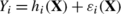(9)

for all i=1,…,m, where the functions $$h_{i}: \mathcal{X} \rightarrow \{0,1\}$$ represent the structural parts of the model and the random variables ε i (x) the stochastic parts. This notation is commonly used in multivariate regression (Hastie et al. 2007, Chap. 3.2.4), a problem quite similar to MLC. The main difference between multivariate regression and MLC concerns the type of output, which is real-valued in the former and binary in the latter. A standard assumption of multivariate regression, namely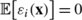(10)

for all $$\mathbf{x} \in\mathcal{X}$$ and i=1,…,m, is therefore not reasonable in MLC.

In general, the distribution of the noise terms can depend on x. Moreover, two noise terms ε i and ε j can also depend on each other, as also the structural parts of the model, say h i and h j , may share some similarities between each other. From this, we can find that there are two possible sources of label dependence: the structural part of the model h(⋅) and the stochastic part ε(⋅).

It seems that marginal dependence between labels is caused by the similarity between the structural parts h i (⋅), simply because one can reasonably assume that the structural part will dominate the stochastic part. Roughly speaking, if there is a function f(⋅) such that h i fh j , meaning that

$$h_i(\mathbf{x}) = f\bigl(h_j(\mathbf{x}) \bigr) + g(\mathbf{x}),$$
(11)

with g(⋅) being “negligible” in the sense that g(x)=0 with high probability (i.e., for most x), then this “f-dependence” between h i and h j is likely to dominate the averaging process in (8), whereas g(⋅) and the error terms ε i will play a less important role (or simply cancel out). This is the case, for example, when the Bayes error rate of the classifiers is relatively low. In other words, the dependence between h i and h j , despite being only probable and approximate, will induce a dependence between the labels Y i and Y j .

### Example 3

Consider a simple problem with a two-dimensional input x=(x 1,x 2) uniformly distributed in [−1,+1]×[−1,+1], and two labels Y 1,Y 2 distributed as follows. The first label is set to one for positive values of x 1, and to zero for negative values, i.e., Y 1=[[x 1>0]].Footnote 1 The second label is defined in the same way, but the decision boundary (x 1=0) is rotated by an angle α∈[0,π]. The two decision boundaries partition the input space into four regions C ij identified by i=Y 1 and j=Y 2. Moreover, the two error terms shall be independent and both flip the label with a probability 0.1 (i.e., ε 1=0 with probability 0.9 and ε 1=1−2[[x 1>0]] with probability 0.1); see Fig. 1 for a typical dataset. For α close to 0, the two labels are almost identical, so a high correlation will be observed, whereas for α=π, they are orthogonal to each other, resulting in a low correlation. More specifically, (11) holds with f(⋅) the identity and g(x) given by ±1 in the “overlap regions” C 01 and C 10 (shaded in gray) and 0 otherwise.

From this point of view, marginal dependence can be seen as a kind of (soft) constraint that a learning algorithm can exploit for the purpose of regularization. This way, it may indeed help to improve predictive accuracy, as will be shown in subsequent sections.

On the other hand, it seems that the stochastic part of the model ε i (⋅) is responsible for the conditional dependence. The posterior probability distribution P(Y|x) provides a convenient point of departure for analyzing conditional label dependence, since it informs about the probability of each label combination as well as the marginal probabilities. In a stochastic sense, as defined above, there is a dependency between the labels if the joint conditional distribution is not the product of the marginals. For instance, in our example above, conditional independence between Y 1 and Y 2 follows from the assumption of independent error terms ε 1 and ε 2. This independence is lost, however, when assuming a close dependency between the error terms, for example ε 1=ε 2. In fact, even though the marginals will remain the same, the joint distribution will change in that case. The following table compares the two distributions for an instance x from the region C 11:

To make a connection to our model (9) we have to define the error terms ε i (⋅) in a proper way. In terms of their expectation, we have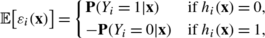for i=1,…,m and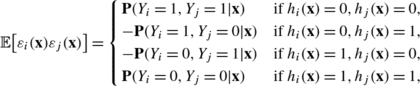for i,j=1,…,m. This observation implies the following proposition that directly links the stochastic part of the model with conditional dependence.

### Proposition 1

A vector of labels (4) is conditionally dependent given x if and only if the error terms in (9) are conditionally dependent given x, i.e.,

$$\mathbb{E}\bigl[\varepsilon _1(\mathbf{x}) \times\cdots\times \varepsilon _m(\mathbf {x})\bigr] \ne\mathbb{E}\bigl[\varepsilon _1( \mathbf{x})\bigr] \cdots \mathbb{E}\bigl[\varepsilon _m(\mathbf{x})\bigr].$$

### Proof

When conditioning on a given input x, one can write Y i =q(ε i ) with q a function. Independence of the error terms then implies independence of the labels. The reverse statement also holds because h becomes a constant for a given x. □

A less general statement has been put forward in Dembczyński et al. (2010b), and independently in Zhang and Zhang (2010).

Let us also underline that conditional dependence may cause marginal dependence, because of (8). In other words, the similarity between the models is not the only source of the marginal dependence.

Briefly summarized, one will encounter conditional dependence between labels if dependencies are observed in the errors terms of the model. On the other hand, the observation of label correlations in the training data will not necessarily imply any dependence between error terms. Label correlations only provide evidence for the existence of marginal dependence between labels, even though the conditional dependence might be a cause of this dependence.

In the remainder of this paper, we will address the idea of exploiting label dependence in learning multi-label classifiers in more detail. We will claim that exploiting both types of dependence, marginal and conditional, can in principle improve the generalization performance, but the true benefit does also depend on the particular formulation of the problem. Furthermore, we will also argue that some of the existing algorithms are interpreted in a somewhat misleading way.

## Three views on multi-label classification

In this section, a link between label dependence and loss minimization is established. As will be seen, this link follows quite naturally, since the discussion of the dependence of error terms boils down to a discussion about loss functions. Moreover, the existence of multiple labels suggests to look at the learning problem from different perspectives. In terms of loss minimization, we distinguish three views, each of them being determined by the type of loss function to be minimized, the type of dependence taken into account, and the distinction between marginal and joint distribution estimation.

1. 1.

The “individual label” view: How can we improve the predictive accuracy of a single label by using information about other labels? Moreover, what are the requirements for improvement? (This view is closely connected to transfer and multi-task learning (Caruana 1997).)

2. 2.

The “joint label” view: What type of proper (non-decomposable) MLC loss functions is suitable for evaluating a multi-label prediction as a whole, and how to minimize such loss functions?

3. 3.

The “joint distribution” view: Under what conditions is it reasonable (or even necessary) to estimate the joint conditional probability distribution over all label combinations?

### Improving single label predictions

Let us first analyze the following question: Can we improve the predictive accuracy for a single label by using the information about other labels? In other words, the question is whether we can improve the binary relevance approach by exploiting relationships between labels. We will refer to this scenario as single label predictions.

More generally, the question relates to problems in which the goal is to minimize a loss function that is label-wise decomposable. The simplest loss function of this type is Hamming loss, which is defined as the fraction of labels whose relevance is incorrectly predicted:

$$L_H\bigl(\mathbf{y}, \mathbf{h}( \mathbf{x})\bigr) = \frac{1}{m} \sum_{i=1}^m \bigl[\!\!\bigl[ y_i \neq h_i(\mathbf{x}) \bigr]\!\!\bigr].$$
(12)

For the Hamming loss (12), it is easy to see that the risk minimizer (3) is obtained by

$$\mathbf{h}^*_H(\mathbf{x}) = \bigl(h_{H_1}(\mathbf{x}), \ldots, h_{H_m}(\mathbf{x})\bigr),$$

where

$$h_{H_i}(\mathbf{x}) = \arg\max_{b \in\{0,1\}} \mathbf {P}(Y_i = b | \mathbf{x} ) \quad(i = 1,\ldots, m).$$
(13)

From this simple analysis, we can conclude that it is enough to take the marginal (single-label) distribution P(Y i |x) into account in order to solve the problem.Footnote 2 At least this is true on the population level, assuming that the hypothesis space is unconstrained. An even stronger result has been obtained in multivariate regression, where one usually minimizes the squared-error label-wise:

$$L_2\bigl(\mathbf{y}, \mathbf{h}(\mathbf{x}) \bigr) = \frac{1}{m} \sum_{i=1}^m \bigl(y_i - h_i(\mathbf{x})\bigr)^2.$$
(14)

In this case, the components of the risk minimizing vector $$\mathbf {h}^{*}_{2}(\mathbf{x})$$ take the form

$$h_{2_i}(\mathbf{x}) = \mathbb{E}(Y_i | \mathbf{x} ) \quad(i = 1,\ldots, m).$$
(15)

A classical result states that linear models obtained by ordinary least squares are the same, regardless of whether the outputs are treated jointly or independently of each other. This remains true even when the inverse of the covariance matrix is involved in the squared-error loss (Hastie et al. 2007, Chap. 3.2.4). Fortunately, as will be seen later on, there are nevertheless possibilities to improve predictive performance. First, however, let us discuss some other loss functions that fall into this scenario.

In general, any loss function for binary classification can be used in MLC, by averaging the losses over the labels:

$$L_M = \frac{1}{m} \sum _{i=1}^m \bar{L}_i\bigl((y_{i1}, y_{i2}, \ldots, y_{in}), (\hat{y}_{i1}, \hat{y}_{i2}, \ldots, \hat{y}_{in})\bigr)$$
(16)

$$\bar{L}_{i}$$ is a loss function that evaluates the predictions for the i-th label on the test set (y ij indicates the presence of the i-th label in the j-th example, and $$\hat{y}_{ij}$$ is the prediction of this value). Obviously, $$\bar{L}_{i}$$ may correspond to the average misclassification or squared-error loss over the examples, leading eventually to the same results as for (12) and (14), respectively. Note, however, that the loss (16) is more general in the sense that it does not assume $$\bar{L}_{i}$$ to decompose linearly into a sum of losses on individual examples. Thus, it also covers measures such as AUC and F-measure.

Let us also mention that (16) is usually referred to as the macro-average as the performance is averaged over single labels, thus attributing equal weights to the labels. In contrast, the micro-average, also commonly used in MLC, gives equal weights to all classifications as it is computed over all predictions simultaneously, for example, by first summing contingency matrices for all labels and then computing the performance over the resulting global contingency matrix (only one matrix). However, this kind of averaging does not fall into any of the views considered in this paper. In the next subsection, we discuss, in turn, losses that are decomposable over single instances.

Our discussion so far implies that the single label prediction problem can be solved on the basis of the marginal distributions P(Y i |x) alone. Hence, with a proper choice of base classifiers and parameters for estimating the marginal probabilities, there is in principle no need for modeling conditional dependence between the labels. This does not exclude the possibility of first modeling the conditional joint distribution (so, conditional dependencies as well) and then perform a proper marginalization procedure. We discuss such an approach in Sect. 4.3. Here in turn, we take a closer look at another possibility that relies on exploiting marginal dependence.

As mentioned in the previous section, marginal dependence is often caused by similarity between the structural parts of the model. Consider an extreme situation in which two models share the same structural part h(x) (a similar example is given in Hastie et al. 2007, Chap. 3.7) in the context of multivariate regression):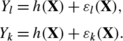Remark that Example 3 represents such a situation when α=0. In this case, the training examples for Y k and Y l can be pooled into a single dataset of double size, thereby decreasing the variance in estimating the parameters of h. The same can of course also be done in cases where the structural parts are only approximately identical. Then, however, a bias will be introduced, and a gain can only be achieved if this negative effect will be dominated by the positive effect, namely the reduction in variance.

In Sect. 6, we will discuss some existing MLC algorithms that improve the performance measured in terms of label-wise decomposable loss functions by exploiting the similarities between the structural parts of the models. Here, let us only add that similarity between structural parts can also be seen as a specific type of background knowledge of the form h l (x)=f(h k (x)), i.e., knowledge about a functional dependence between the deterministic parts of the models for individual labels. Given a label-wise decomposable loss function, an improvement over BR can also be achieved by using any sort of prior knowledge about the marginal dependence between the labels.

### Minimization of multi-label loss functions

In the framework of MLC, one can consider a multitude of loss functions. We have already discussed the group of losses that are decomposable over single labels, i.e., losses that can be represented as an average over labels. Here, we discuss loss functions that are not decomposable over single labels, but decomposable over single instances. Particularly, we focus on rank loss, F-measure loss, Jaccard distance, and subset 0/1 loss. We start our discussion with the rank loss by showing that this loss function is still closely related to single label predictions. Later, we will discuss the subset 0/1 loss, which is in turn closely related to the estimation of the joint probability distribution. The two remaining loss functions, F-measure loss and Jaccard distance, are more difficult to analyze, and there is no easy way to train a classifier minimizing them.

Let us assume that the true labels constitute a ranking in which all relevant labels (i.e., those with y i =1) ideally precede all irrelevant ones (y i =0), and h is a ranking function representing a degree of label relevance sorted in a decreasing order. The rank loss simply counts the number of label pairs that disagree in these two rankings:

$$L_r\bigl(\mathbf{y},\mathbf{h}( \mathbf{x})\bigr) = \sum_{(i,j): y_i > y_j} \biggl( \bigl[\!\!\bigl[ h_i(\mathbf{x}) < h_j(\mathbf{x}) \bigr]\!\!\bigr] + \frac{1}{2} \bigl[\!\!\bigl[ h_i(\mathbf{x}) = h_j(\mathbf{x}) \bigr]\!\!\bigr] \biggr).$$
(17)

Since this loss function is neither convex nor differentiable, a common approach is to minimize a convex surrogate in which the boolean predicate is substituted by the hinge (like in SVM) or exponential (like in boosting) function. Nevertheless, to minimize (17), it is enough to sort the labels by their probability of relevance. Formally, we can show the following result (the proof is given in the Appendix).

### Theorem 1

A ranking function that sorts the labels according to their probability of relevance, i.e., using the scoring function h(⋅) with

$$h_i(\mathbf{x}) = \mathbf {P}(Y_i = 1 | \mathbf{x}),$$
(18)

minimizes the expected rank loss (17).

As one of the most important consequences of the above result we note that, according to (18), a risk-minimizing prediction for the rank loss can be obtained from the marginal distributions P(Y i |x) (i=1,…,m) alone. Thus, just like in the case of Hamming loss, it is in principle not necessary to know the joint label distribution P(Y|x) on $$\mathcal{Y}$$, which means that risk-minimizing predictions can be made without any knowledge about the conditional dependency between labels. In other words, this result suggests that instead of minimizing the rank loss directly, one can simply use any approach for single label prediction that properly estimates the marginal probabilities.

In passing, we note that there is also a normalized variant of the rank loss, in which the number of mistakes is divided by the maximum number of possible mistakes on y, i.e., by the number of summands in (17); this number is given by r(mr)/2, with $$r= \sum_{i=1}^{m} y_{i}$$ the number of relevant labels. Without going into detail, we mention that the above result cannot be extended to the normalized version of the rank loss. That is, knowing the marginal distributions P(Y i  | x) is not enough to produce a risk minimizer in this case.

The next multi-label loss function we analyze is the subset 0/1 loss, which generalizes the well-known 0/1 loss from the conventional to the multi-label setting:

$$L_s\bigl(\mathbf{y}, \mathbf{h}(\mathbf{x})\bigr) = \bigl[\!\!\bigl[\mathbf{y} \ne\mathbf {h}(\mathbf{x}) \bigr]\!\!\bigr].$$
(19)

Admittedly, this loss function may appear overly stringent, especially in the case of many labels. Moreover, since making a mistake on a single label is punished as hardly as a mistake on all labels, it does not discriminate well between “almost correct” and “completely wrong” predictions. Still, as will be seen next, this measure is obviously interesting with regard to label dependence.

As for any other 0/1 loss, the risk-minimizing prediction for (19) is simply given by the mode of the distribution:

$$\mathbf{h}^*_s(\mathbf{x}) = \arg\max_{\mathbf{y} \in\mathcal{Y}} \mathbf {P}(\mathbf {y} | \mathbf{x}).$$
(20)

In contrast to the result for the rank loss, (20) shows that the entire distribution of Y given X, or at least enough knowledge to identify the mode of this distribution, is needed to minimize the subset 0/1 loss. In other words, the derivation of a risk-minimizing prediction requires the modeling of the joint distribution (at least to some extent), and hence the modeling of conditional dependence between labels.

Finally, let us have a look at losses based on the F-measure and the Jaccard distance between sets. In the previous subsection, we already mentioned the F-measure loss, but we computed it for each label independently. In contrast, the instance-wise decomposable version is defined over all labels simultaneously:Footnote 3

$$L_F\bigl(\mathbf{y}, \mathbf{h}(\mathbf{x})\bigr) = 1 - \frac{2 \sum_{i=1}^m y_i h_i(\mathbf{x})}{\sum_{i=1}^m y_i + \sum_{i=1}^m h_i(\mathbf{x})},$$
(21)

where we assume that h i (x)∈{0,1}. This measure can also be defined as the harmonic mean of precision and recall computed for a single instance.

The Jaccard distance is quite similar to the F-measure loss, but it is originally defined by set operators as one minus the ratio of intersection and union:

$$L_J\bigl(\mathbf{y}, \mathbf{h}(\mathbf{x})\bigr) = 1 - \frac{ |\{i \mid y_i = 1 \wedge h_i = 1, i=1,\ldots,m \}|}{| \{i \mid y_i = 1 \vee h_i = 1, i=1,\ldots ,m \}|}.$$
(22)

Thanks to some simple transformations, it can also be written as follows:

$$L_J \bigl(\mathbf{y}, \mathbf{h}(\mathbf{x})\bigr) = 1 - \frac{\sum_{i=1}^m y_i h_i(\mathbf {x})}{\sum_{i=1}^m y_i + \sum_{i=1}^m h_i(\mathbf{x}) - \sum_{i=1}^m y_i h_i(\mathbf{x})}.$$
(23)

It is an open question whether or not a closed-form solution for the risk minimizers of these loss functions exists. Moreover, the minimization of them is not straightforward. In a recent paper, we show that the F-measure loss can be minimized in an efficient manner using m 2+1 parameters of the conditional joint distribution over labels (Dembczyński et al. 2012). For the Jaccard index, one commonly believes that exact optimization is much harder (Chierichetti et al. 2010).

### Conditional joint distribution estimation

The last view on MLC problems discussed in this paper concerns the estimation of the joint probability distribution P(Y|X). Estimating this distribution can be useful for several reasons. For example, we have shown that the joint mode is the risk-minimizer of the subset 0/1 loss, and one way to obtain this value is through modeling the joint distribution. More generally, if the joint distribution is known, a risk-minimizing prediction can be derived for any loss function L(⋅) in an explicit way:

$$\mathbf{h}^*(\mathbf{x}) = \arg\min_{\mathbf{y}} \mathbb{E}_{\mathbf{Y}|\mathbf {x}} L( \mathbf{Y},\mathbf{y}).$$

This also applies for loss functions for which a solution can be solely obtained from marginal probabilities. In some applications modeling the joint distribution may result in using simpler classifiers, potentially leading to a lower cost and a better performance compared to directly estimating marginal probabilities by means of more complex classifiers.

Nevertheless, the estimation of the joint probability is a difficult task. In general one has to estimate 2m values for a given x, namely the probability degrees P(y|x) for all $$\mathbf{y} \in\mathcal{Y}$$. In order to solve this problem efficiently, all methods for probability estimation can in principle be used. This includes parametric approaches based on Gaussian distributions or exponential families, reducing the problem to the estimation of a small number of parameters (Joe 2000). It also includes graphical models such as Bayesian networks (Jordan 1998), which factorize a high-dimensional distribution into the product of several lower-dimensional distributions. For example, departing from the product rule of probability (7), one can try to simplify a joint distribution by exploiting label independence whenever possible, ending up with (6), in the extreme case of conditional independence.

As another useful tool for modeling a joint distribution, which appears to be especially interesting in the context of MLC, we mention so-called copulas. Copulas are functions with certain well-defined properties that characterize the dependence of random variables by establishing a link between marginal cumulative and joint cumulative distribution functions. Although the early work on copulas dates back to the 50s of the last century, these functions have received increasing attention in statistics and several applied disciplines in the last years. The main result given by Sklar (1959) states that for an m-dimensional distribution function F with marginal distribution functions F 1,F 2,…,F m , there exists an m-copula C:[0,1]m→[0,1] such that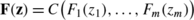for all z in ℝm. An m-copula can be interpreted as the joint cumulative density function of a set of m random variables defined on the interval [0,1].

To the best of our knowledge, copulas have not been used in MLC so far, although they suggest a natural two-step procedure for estimating joint conditional distributions:

• First, obtain estimates of the conditional marginal distributions for every label separately. This step could be considered as a probabilistic binary relevance approach.

• Subsequently, estimate a copula on top of the marginal distributions to obtain the conditional joint distribution.

Such a procedure is common practice in statistics, usually not for predictive purposes, but mainly to gain deeper insight into the dependence between different labels (Joe 2000). Notwithstanding the potential merits of such approaches in a purely predictive MLC setting, two important limitations of existing work should be observed. First, these approaches are highly parametric; typically the parameters of Gaussian copulas are estimated. Second, the existence of one global copula is assumed, irrespective of x.

## Theoretical insights into multi-label classification

In many MLC papers, a new learning algorithm is introduced without clearly stating the problem to be solved. Then, the algorithm is empirically tested with respect to a multitude of performance measures, but without precise information about which of these measures the algorithm actually intends to optimize. This may implicitly give the misleading impression that the same method can be optimal for several loss functions at the same time.

In this section, we provide theoretical evidence for the claim that our distinction between MLC problems, as proposed in the previous section, is indeed important. A classifier supposed to be good for solving one of those problems may perform poorly for another problem. In order to facilitate the analysis, we restrict ourselves to two loss functions, namely the Hamming and the subset 0/1 loss. The first one is representative of the single label scenario, while the second one is a typical multi-label loss function whose minimization calls for an estimation of the joint distribution. Our analysis proceeds from the simplifying assumption of an unconstrained hypothesis space, which allows us to consider the conditional distribution for a given x. As such, this theoretical analysis will differ from the experimental analysis reported in Sect. 7, where parametric hypothesis spaces are considered. Despite this conceptual difference, our theoretical and experimental results will be highly consistent. They both support the main claims of this paper concerning loss minimization and its relationship with label dependence. While the theoretical analysis mainly provides evidence on the population level, the empirical study also investigates the effect of estimation.

The main result of this section will show that, in general, the Hamming loss minimizer and the subset 0/1 loss minimizer will differ significantly. That is, the Hamming loss minimizer may be poor in terms of the subset 0/1 loss and vice versa. In some (not necessarily unrealistic) situations, however, the Hamming and subset 0/1 loss minimizers coincide, an observation that may explain some misleading results in recent MLC papers. The following proposition reveals two such situations.

### Proposition 2

The Hamming loss and subset 0/1 have the same risk minimizer, i.e., $$\mathbf{h}^{*}_{H}(\mathbf{x}) = \mathbf{h}^{*}_{s}(\mathbf{x})$$, if one of the following conditions holds:

1. (1)

Labels Y 1,…,Y m are conditionally independent, i.e., $$\mathbf {P}(\mathbf{Y}|\mathbf{x}) = \prod_{i=1}^{m} \mathbf {P}(Y_{i}|\mathbf{x})$$.

2. (2)

The probability of the mode of the joint probability is greater than or equal to 0.5, i.e., $$\mathbf {P}(\mathbf{h}^{*}_{s}(\mathbf{x})|\mathbf {x}) \ge0.5$$.

### Proof

1. (1)

Since the joint probability of any combination of y is given by the product of marginal probabilities, the highest value of this product is given by the highest values of the marginal probabilities. Thus, the joint mode is composed of the marginal modes.

2. (2)

If $$\mathbf {P}(\mathbf{h}^{*}_{s}(\mathbf{x})|\mathbf{x}) \ge0.5$$, then $$\mathbf {P}(h^{*}_{s_{i}}(\mathbf{x})|\mathbf{x}) \ge0.5$$, i=1,…,m, and from this it follows that $$h^{*}_{s_{i}}(\mathbf{x}) = h^{*}_{H_{i}}(\mathbf{x})$$.

□

As a simple corollary of this proposition, we have the following.

### Corollary 1

In the separable case (i.e., the joint conditional distribution is deterministic, P(Y|x)=[[Y=y]], where y is a binary vector of size m), the risk minimizers of the Hamming loss and subset 0/1 coincide.

### Proof

If P(Y|x)=[[Y=y]], then $$\mathbf {P}(\mathbf{Y}|\mathbf{x}) = \prod_{i=1}^{m} \mathbf {P}(Y_{i}|\mathbf{x})$$. In this case, we also have $$\mathbf {P}(\mathbf{h}^{*}_{s}(\mathbf{x})|\mathbf{x}) \ge0.5$$. Thus, the result follows from both (1) and (2) in Proposition 2. □

Moreover, one can claim that the two loss functions are related to each other because of the following simple bounds (the proof is given in the Appendix).

### Proposition 3

For all distributions of Y given x, and for all models h, the expectation of the subset 0/1 loss can be bounded in terms of the expectation of the Hamming loss as follows: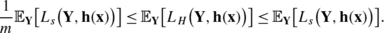However, the next result shows that using a classifier tailored for the wrong loss function may yield a high discrepancy in performance. We define the regret of a classifier h with respect to a loss function L z as follows:

$$r_{L_z}(\mathbf{h}) = R_{L_z}(\mathbf{h}) - R_{L_z}\bigl(\mathbf{h}^*_z\bigr),$$
(24)

where R is the risk given by (1), and $$\mathbf{h}^{*}_{z}$$ is the Bayes-optimal classifier with respect to the loss function L z .

In the following, we consider the regret with respect to the Hamming loss, given by

$$r_H(\mathbf{h}) = \mathbb{E}_{\mathbf{X}\mathbf{Y}} L_H\bigl( \mathbf{Y}, \mathbf{h}(\mathbf{X})\bigr) - \mathbb{E}_{\mathbf{X}\mathbf{Y}} L_H\bigl(\mathbf {Y}, \mathbf{h}^*_H(\mathbf{X})\bigr),$$

and the subset 0/1 loss, given by

$$r_s(\mathbf{h}) = \mathbb{E}_{\mathbf{X}\mathbf{Y}} L_s\bigl( \mathbf{Y}, \mathbf{h}(\mathbf{X})\bigr) - \mathbb{E}_{\mathbf{X}\mathbf{Y}} L_s\bigl(\mathbf {Y}, \mathbf{h}^*_s(\mathbf{X})\bigr).$$

Since both loss functions are decomposable with respect to individual instances, we analyze the expectation over Y for a given x. The first result concerns the highest value of the regret in terms of the subset 0/1 loss for $$\mathbf{h}^{*}_{H}(\mathbf{X})$$, the optimal strategy for the Hamming loss (the proof is given in the Appendix).

### Proposition 4

The following upper bound holds:

$$\mathbb{E}_{\mathbf{Y}} L_s\bigl(\mathbf{Y}, \mathbf{h}^*_H(\mathbf{x})\bigr) - \mathbb{E}_{\mathbf{Y}} L_s\bigl(\mathbf{Y}, \mathbf{h}^*_s(\mathbf{x})\bigr) < 0.5.$$

Moreover, this bound is tight, i.e.,

$$\sup_{\mathbf {P}} \bigl(\mathbb{E}_{\mathbf{Y}} L_s\bigl( \mathbf{Y}, \mathbf {h}^*_H(\mathbf{x})\bigr) - \mathbb{E}_{\mathbf{Y}} L_s\bigl(\mathbf{Y}, \mathbf {h}^*_s(\mathbf{x})\bigr) \bigr) = 0.5,$$

where the supremum is taken over all probability distributions on $$\mathcal{Y}$$.

The second result concerns the highest value of the regret in terms of the Hamming loss for $$\mathbf{h}^{*}_{s}(\mathbf{X})$$, the optimal strategy for the subset 0/1 loss (the proof is given in the Appendix).

### Proposition 5

The following upper bound holds for m>3:

$$\mathbb{E}_{\mathbf{Y}} L_H\bigl(\mathbf{Y}, \mathbf{h}^*_s(\mathbf{x})\bigr) - \mathbb{E}_{\mathbf{Y}} L_H\bigl(\mathbf{Y}, \mathbf{h}^*_H(\mathbf{x})\bigr) < \frac{m-2}{m+2}.$$

Moreover, this bound is tight, i.e.

$$\sup_{\mathbf {P}}\bigl(\mathbb{E}_{\mathbf{Y}} L_H\bigl( \mathbf{Y}, \mathbf{h}^*_s(\mathbf {x})\bigr) - \mathbb{E}_{\mathbf{Y}} L_H\bigl(\mathbf{Y}, \mathbf{h}^*_H(\mathbf{x})\bigr) \bigr) = \frac{m-2}{m+2},$$

where the supremum is taken over all probability distributions on $$\mathcal{Y}$$.

As we can see, the worst case regret is high for both loss functions, suggesting that a single classifier will not be able to perform equally well in terms of both functions. Instead, a classifier specifically tailored for the Hamming (subset 0/1) loss will indeed perform much better for this loss than a classifier trained to minimize the subset 0/1 (Hamming) loss.

## MLC algorithms for exploiting label dependence

Recently, a number of learning algorithms for MLC have been proposed in the literature, mostly with the goal to improve predictive performance (in comparison to binary relevance learning), but sometimes also having other objectives in mind (e.g., reduction of time complexity (Hsu et al. 2009)). To achieve their goals, the algorithms typically seek to exploit dependencies between the labels. However, as mentioned before, concrete information about the type of dependency tackled or the loss function to be minimized is rarely given. In many cases, this is a cause of confusion and ill-designed experimental studies, in which inappropriate algorithms are used as baselines.

Tsoumakas and Katakis (2007) distinguish two categories of MLC algorithms, namely problem transformation methods (reduction) and algorithm adaptation methods (adaptation). Here, we focus on algorithms from the first group, mainly because they are simple and widely used in empirical studies. Thus, a proper interpretation of these algorithms is strongly desired.

We discuss reduction algorithms in light of our three views on MLC problems. We will start with a short description of the BR approach. Then, we will present algorithms being tailored for single label predictions by exploiting the similarities between structural parts of the models. Next, we will discuss algorithms taking into account conditional label dependence, and hence being tailored for other multi-label loss functions, like the subset 0/1 loss. Some of these algorithms are also able to estimate the joint distribution. To summarize the discussion on these algorithms we present their main properties in a table. Let us, however, underline that this description concerns the basic settings of these algorithms given in the original papers. It may happen that one can extend their functionality by alternating their setup. At the end of this section, we give a short review of adaptation algorithms, but their detailed description is beyond the scope of this paper. We also shortly describe algorithms devoted for multi-label ranking problems.

### Binary relevance

As we mentioned before, BR is the simplest approach to multi-label classification. It reduces the problem to binary classification, by training a separate binary classifier h i (⋅) for each label λ i . Learning is performed independently for each label, ignoring all other labels.

Obviously, BR does not take label dependence into account, neither conditional nor marginal. Indeed, as suggested by our theoretical results, BR is, in general, not able to yield risk minimizing predictions for losses like subset 0/1, but it is well-tailored for Hamming loss minimization or, more generally, every loss whose risk minimizer can be expressed solely in terms of marginal distributions P(Y i |x) (i=1,…,m). As confirmed by several experimental studies, this approach might be sufficient for getting good results in such cases. However, exploiting marginal dependencies may still be beneficial, especially for small-sized problems.

### Single label predictions

There are several methods that exploit similarities between the structural parts of label models. The general scheme of these approaches can be expressed as follows:

$$\mathbf{y} = \mathbf{b} \bigl(\mathbf{h}(\mathbf{x}), \mathbf{x}\bigr),$$
(25)

where h(x) is the binary relevance learner, and b(⋅) is an additional classifier that shrinks or regularizes the solution of BR. One can also consider a slightly modified scheme:

$$\mathbf{b}^{-1}(\mathbf{y}, \mathbf{x}) = \mathbf{h}(\mathbf{x}).$$
(26)

In this case, the output space (possibly along with the feature space) is first transformed, and the binary relevance classifiers (or rather regressors, since the domain of the transformed outputs is usually a set of real numbers) are then trained on the new output variables b −1(y,x). Finally, to obtain a prediction of the original variables, the inverse transform has to be performed, usually along with a kind of shrinkage/regularization.Footnote 4

### Stacking.

Methods like Stacking (Godbole and Sarawagi 2004; Cheng and Hüllermeier 2009) directly follow the first scheme (25). They replace the original predictions, obtained by learning every label separately, by correcting them in light of information about the predictions of the other labels. This transformation of the initial prediction should be interpreted as a regularization procedure. Another possible interpretation is a feature expansion. This method can easily be used with any kind of binary classifier. It is not clear, in general, whether the meta-classifier b should be trained on the BR predictions h(x) alone or use the original features x as additional inputs. Another question concerns the type of information provided by the BR predictions. One can use binary predictions, but also values of scoring functions or probabilities, if such outputs are delivered by the classifier.

### Multivariate regression.

Several methods introduced for multivariate regression, like C&W (Breiman and Friedman 1997), reduced-rank regression (RRR) (Izenman 1975), and FICYREG (an der Merwe and Zidek 1980), can be seen as a realization of the scheme (25). According to Breiman and Friedman (1997), these methods have the same generic form:

$$\mathbf{y} = \bigl(\mathbf{T}^{\mathbf{-1}}\mathbf{GT}\bigr)\mathbf{A}\mathbf{x},$$

where T is the matrix of sample canonical co-ordinates, the solution of the canonical correlation analysis (CCA), and the diagonal matrix G contains the shrinkage factors for scaling the solutions of ordinary linear regression A.

These methods can also be represented by the second scheme (26). First, y is transformed to the canonical co-ordinate system y′=Ty. Then, separate linear regression is performed to obtain estimates $$\tilde{\mathbf{y}}' = (\tilde{y}'_{1}, \tilde{y}'_{2}, \ldots, \tilde{y}'_{n})$$. These estimates are further shrunk by the factor g ii obtaining $$\hat{\mathbf{y}}' = \mathbf{G} \tilde{\mathbf{y}}'$$. Finally, the prediction is transformed back to the original co-ordinate output space $$\hat{\mathbf{y}} = \mathbf {T}^{-1} \hat{\mathbf{y}}'$$.

### Kernel dependency estimation.

The above references rather originate from the statistics domain, but similar approaches have also been introduced in machine learning, like kernel dependency estimation (KDE) (Weston et al. 2002) and multi-output regularized feature projection (MORP) (Yu et al. 2006). We focus here on the former method. It consists of a three-step procedure. The first step conducts a kernel principal component analysis of the label space for deriving non-linear combinations of the labels or for predicting structured outputs. Subsequently, the transformed labels (i.e., the principal components) are used in a simple multivariate regression method that does not have to care about label dependencies, knowing that the transformed labels are uncorrelated. In the last step, the predicted labels of test data are transformed back to the original label space. Since Kernel PCA is used, this transformation is not straightforward, and the so-called pre-image problem has to be solved. Label-based regularization can be included in this approach as well, simply by using only the first r<m principal components in steps two and three, similar to regularization based on feature selection in methods like principal component regression (Hastie et al. 2007). The main difference between KDE and multivariate regression methods described above is the use of kernel PCA instead of CCA. Simplified KDE approaches based on PCA have been studied for multi-label classification in Tai and Lin (2010). Here, the main goal was to reduce the computational costs by using only the most important principal components.

### Compressive sensing.

The idea behind compressive sensing used for MLC (Hsu et al. 2009) is quite different, but the resulting method shares a lot of similarities with the algorithms described above. The method assumes that the label sets can be compressed and we can learn to predict the compressed labels instead. From this point of view, we can mainly improve the time complexity, since we solve a lower number of core problems. The compression of the label sets is possible only if the vectors y are sparse. This method follows scheme (26) to some extent. The main difference is the interpretation of the matrix T. Here, we obtain y′=Ty by using a random matrix from an appropriate distribution (such as Gaussian, Bernoulli, or Hadamard) whose number of rows is much smaller than the length of y. This results in a new multivariate regression problem with a lower number of outputs. The prediction for a novel x relies on computing the output of the regression problem $$\hat{\mathbf{y}}'$$, and then on obtaining a sparse vector $$\hat{\mathbf{y}}$$ such that $$\mathbf{T}\hat{\mathbf {y}}'$$ is closest to $$\hat{\mathbf{y}}'$$ solving an optimization problem, similarly as in KDE. In other words, there is no simple decoding from the compressed to the original label space, as it was the case for multivariate regression methods.

### Estimation of joint distribution and minimization of multi-label loss functions

Here, we describe some methods that seek to estimate the joint distribution P(Y|x). As explained in Sect. 4.3, knowledge about the joint distribution (or an estimation thereof) allows for an explicit derivation of the risk minimizer of any loss function. However, we also mentioned the high complexity of this approach.

### Label Powerset (LP).

This approach reduces the MLC problem to multi-class classification, considering each label subset $$L \in\mathcal{L}$$ as a distinct meta-class (Tsoumakas and Katakis 2007; Tsoumakas and Vlahavas 2007). The number of these meta-classes may become as large as $$|\mathcal{L}| = 2^{m}$$, although it is often reduced considerably by ignoring label combinations that never occur in the training data. Nevertheless, the large number of classes produced by this reduction is generally seen as the most important drawback of LP.

Since prediction of the most probable meta-class is equivalent to prediction of the mode of the joint label distribution, LP is tailored for the subset 0/1 loss. In the literature, however, it is often claimed to be the right approach to MLC in general, as it obviously takes the label dependence into account. This claim is arguably incorrect and does not discern between the two types of dependence, conditional and unconditional. In fact, LP takes the conditional dependence into account and usually fails for loss functions like Hamming.

Let us notice that LP can easily be extended to any other loss function, provided the underlying multi-class classifier f(⋅) does not only provide a class prediction but a reasonable estimate of the probability of all meta-classes (label combinations), i.e., f(x)≈P(Y|x). From this point of view, LP can be seen as a method for estimating the conditional joint distribution. Practically, however, the large number of meta-classes makes probability estimation an extremely difficult problem. In this regard, we also mention that most implementations of LP essentially ignore label combinations that are not presented in the training set or, stated differently, tend to underestimate (set to 0) their probabilities.

Several extensions of LP have been proposed in order to overcome its computational burden. The RAKEL algorithm (Tsoumakas and Vlahavas 2007) is an ensemble method that consists of several LP classifiers defined on randomly drawn subsets of labels. This method is parametrized by a number of base classifiers and the size of label subsets. A global prediction is obtained by combining the predictions of the ensemble members on the label subsets. Essentially, this is done by counting, for each label, how many times it is included in a predicted label subset. Despite its intuitive appeal and competitive performance, RAKEL is still not well understood from a theoretical point of view. For example, it is not clear what loss function it intends to minimize.

### Probabilistic Classifier Chains (PCC).

The number of meta-classes produced in LP is exponential in the number of labels, which is clearly problematic from a classification point of view. One possibility to circumvent this problem is to predict label combinations in a stepwise manner, label by label, as suggested by the product rule of probability (7):

$$\mathbf {P}(\mathbf{Y}|\mathbf{x}) = \mathbf {P}(Y_1|\mathbf{x}) \prod _{i=2}^{m} \mathbf {P}(Y_i|Y_1, \ldots,Y_{i-1},\mathbf{x}).$$
(27)

More specifically, to estimate the joint distribution of labels, one possibility is to learn m functions g i (⋅) on augmented input spaces $$\mathcal{X} \times\{0,1\}^{i-1}$$, respectively, taking y 1,…,y i−1 as additional attributes: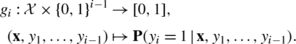Here, we assume that the function g i (⋅) can be interpreted as a probabilistic classifier whose prediction is the probability that y i =1, or at least a reasonable approximation thereof. This approach (Dembczyński et al. 2010a) is referred to as probabilistic classifier chains, or PCC for short. As it essentially comes down to training m binary classifiers (in augmented feature spaces), this approach is manageable from a learning point of view, both conceptually and computationally.

Much more problematic, however, is doing inference from the given joint distribution. In fact, exact inference will again come down to using (27) in order to produce a probability degree for each label combination, and hence cause an exponential complexity. Since this approach is infeasible in general, approximate methods may have to be used. For example, a simple greedy approximation of the joint mode is obtained by successively choosing the most probable label according to each of the classifiers’ predictions. This approach, referred to as classifier chains (CC), has been introduced in Read et al. (2009), albeit without a probabilistic interpretation. Alternatively, one can exploit (27) to sample from it. Then, one can compute a response for a given loss function based on this sample. Such an approach has been used for the F-measure in Dembczyński et al. (2012).

Theoretically, the result of the product rule does not depend on the order of the variables. Practically, however, two different classifier chains will produce different results, simply because they involve different classifiers learned on different training sets. To reduce the influence of the label order, Read et al. (2009) propose to average the multi-label predictions of CC over a (randomly chosen) set of permutations. Thus, the labels λ 1,…,λ m are first re-ordered by a permutation π of {1,…,m}, which moves the label λ i from position i to position π(i), and CC is then applied as usual. This extension is called the ensembled classifier chain (ECC). In ECC, a prediction is made by averaging over several CC predictions. However, like in the case of RAKEL, it is rather unclear what this approach actually tends to estimate, and what loss function it seeks to minimize.

We summarize the main properties of the algorithms described so far in a tabular form. Table 1 gives a simple comparison of the algorithms in terms of loss functions they minimize and the way they model the label dependence.

### Other approaches to MLC

For the sake of completeness, let us mention that the list of methods discussed so far is not exhaustive. In fact, there are several other methods that are potentially interesting in the context of MLC. This includes, for example, conditional random fields (CRF) (Lafferty et al. 2001; Ghamrawi and McCallum 2005), a specific type of graphical model that allows for representing relationships between labels and features in a quite convenient way. This approach is designed for finding the joint mode, thus for minimizing the subset 0/1 loss. It can also be used for estimating the joint probability of label combinations.

Instead of estimating the joint probability distribution, one can also try to minimize a given loss function in a more direct way. Concretely, this can be accomplished within the framework of structural support vector machines (SSVM) (Tsochantaridis et al. 2005); indeed, a multi-label prediction can be seen as a specific type of structured output. Finley and Joachims (2008) and Hariharan et al. (2010) (M3L) tailored this algorithm explicitly to minimize the Hamming loss in MLC problems. Let us also notice that Pletscher et al. (2010) introduced a generalization of SSVMs and CRFs that can be applied for optimizing a variety of MLC loss functions. Yet another approach to direct loss minimization is the use of boosting techniques. In Amit et al. (2007), so-called label covering loss functions are introduced that include Hamming and the subset 0/1 losses as special cases. The authors also propose a learning algorithm suitable for minimizing covering losses, called AdaBoost.LC.

Finally, let us discuss shortly algorithms that have been designed for the problem of label ranking, i.e., MLC problems in which ranking-based performance measures, like the rank loss (17), are of primary interest. One of the first algorithms of this type was BoosTexter (Schapire and Singer 2000), being an adaptation of AdaBoost. This idea has been further generalized to log-linear models by Dekel et al. (2004). Rank-SVM is an instantiation of SVMs that can be applied for this type of problems (Elisseeff and Weston 2002). Ranking by pairwise comparison (Hüllermeier et al. 2008; Fürnkranz et al. 2008) is a reduction method that transform the MLC problem to a quadratic number of binary problems, one for each pair of labels.

## Experimental evidence

To corroborate our theoretical results by means of empirical evidence, this section presents a number of experimental studies, using both synthetic and benchmark data. We constrained the experiment to four reduction algorithms: BR, Stacking (SBR), CC, and LP. We test these methods in terms of Hamming and subset 0/1 loss. First, we investigate the behavior of these methods on synthetic datasets pointing to some important pitfalls often encountered in experimental studies of MLC. Finally, we present some results on benchmark datasets and discuss them in the light of these pitfalls.

We used an implementation of BR and LP from the MULAN package (Tsoumakas et al. 2010),Footnote 5 and the original implementation of CC (Read et al. 2009) from the MEKA package.Footnote 6 We implemented our own code for Stacking that was built upon the code of BR. In the following experiments, we employed linear logistic regression (LR) as a base classifier of the MLC methods, taking the implementation from WEKA (Witten and Frank 2005).Footnote 7 In some experiments, we also used a rule ensemble algorithm, called MLRules,Footnote 8 which can be treated as a non-linear version of logistic regression, as this method trains a linear combination of decision (classification) rules by maximizing the likelihood (Dembczyński et al. 2008). In SBR, we first trained the binary relevance based on LR or MLRules, and subsequently a second LR for every label, in which the predicted labels (in fact, probabilities) of a given instance are used as additional features. In CC, the base classifier was trained for each consecutive label using the precedent labels as additional inputs, and the prediction was computed in a greedy way, as we adopted here the original version of this algorithm (not the probabilistic one). We took the original order of the labels (in one experiment we trained an ensemble of CCs and in this case we randomized the order of labels). In LP we used the 1-vs-1 method to solve the multi-class problem.

For each binary problem being a result of the reduction algorithm, we applied an internal three-fold cross-validation on training data for tuning the regularization parameters of the base learner. We chose for a given binary problem the model with the lowest misclassification error. For LR we used the following set of possible values of the regularization parameter {1000,100,10,1,0.1,0.01,0.001}. For MLRules, we varied the pairs of the number of rules and the shrinkage parameter. The possible values for the number of rules are {5,10,20,50,100,200,500}. We associated the shrinkage parameter with the number of rules by taking respectively the following values {1,1,1,0.5,0.2,0.2,0.1}.

According to this setting and our theoretical claims, BR and SBR should perform well for the Hamming loss, while CC and LP are more appropriate for the subset 0/1 loss.

### Synthetic data

All synthetic data are based on a simple toy model with up to m=25 labels and linear decision boundaries in a two-dimensional input space. The true underlying models are defined as follows: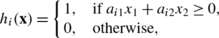with i=1,…,m. Values of x 1 and x 2 were generated according to a unit disk point picking, i.e., uniformly drawn from the circle of the radius equal to 1. Thus, we were not introducing any additional artifact disturbing results of different linear models. Parameters a i =(a i1,a i2) were drawn randomly in order to model different degree of similarity between the labels of a given instance. The labels are similar when the parameters a i are similar, while they tend to be dissimilar if the values are diverse. The parameters a i were controlled by value τ in the following way:

$$a_{i1} = 1 - \tau r_1 , \qquad a_{i2} = \tau r_2,$$

where r 1,r 2U(0,1), i.e., were drawn randomly from the uniform distribution. Next, the parameters were normalized to satisfy ||a i ||2=1. Below we will consider two situations: τ=0, which leads to identical structural parts and a strong marginal dependence; and τ=1, which corresponds to similar but non-identical models and a lower degree of marginal dependence.

In the different experiments, we generated data in several ways based on this simple linear core problem. We varied the similarity of the structural parts of the models, the types of errors and the dependence between them. The training and test sets respectively contained 50 and 10000 instances in all experiments. Each experiment was repeated 100 times to obtain stable results and indications of the variance on the test performance. To this end, error bars are shown in figures presented below. For visualization purposes these error bars are plotted as three times the standard error. In addition, we always generated 10 different models and for each such model we generated 10 different training sets and one test set. Figure 2 shows data points for three exemplary labels with an independent error terms. In the most experiments on synthetic data the linear classifier should be adequate for solving the problems correctly.

### Marginal independence

In this first experiment, the behavior of the MLC methods is analyzed for the case of marginal independence. The problem consists of several linear models as defined above, using τ=1 (in fact, the value of τ does not play any role in this experiment). However, to make the models independent, they were generated in a separate two-dimensional input space: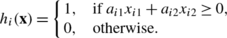Thus, in the case of m labels, the total number of features was then 2m. We tested the performance of the methods varying the number of labels from 1 to 20. Additionally, each label was disturbed by an independent random error term that follows a Bernoulli distribution: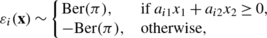in which the Bernoulli parameter π controlled the Bayes error rate for a given subproblem. We chose π=0.1, thereby leading to a Bayes error of π for the Hamming loss and a Bayes error of 1−(1−π)m for the subset 0/1 loss. For large m, the subset 0/1 loss tends to 1.

The error curves are presented in Fig. 3. The lines for the Bayes error are also plotted. Since the labels are completely independent, we can see that Stacking does not improve over BR and instead even obtains worse results. CC performs similarly to SBR, but LP is not able to get good results, probably because of the large number of different label combinations. We can also observe that the error increases with the number of labels. This is probably caused by an increasing number of irrelevant features for a given label. Let us notice, however, that the Hamming loss and the subset 0/1 loss minimizers coincide for this data.

### Conditional independence

In this experiment, we analyze the case of conditional independence. In this case, we used only two features and each label was computed on them using different linear models, in contrast to the previous experiment, where two separate features were constructed for each label individually. The error terms for different labels were independently sampled. They followed a Bernoulli distribution as before with π=0.1. First, we generated data for τ=0. This results in models sharing the same structural part and differing in the stochastic part only. Later, we changed τ to 1. In this case some of the labels can still share some similarities. We can observe marginal dependence, but it is not so extreme as in the previous case. Let us also notice that in this case the risk minimizers for both loss functions coincide.

Figure 4 summarizes the main results obtained in this experiment. One can see that Stacking improves over BR in both cases, but the improvement is higher when the structural parts of the model are identical. This supports our theoretical claim that, the higher the similarity between models for different labels, the more prominent the effect of Stacking. For the Hamming loss, one can observe that the performance of SBR slightly increases to some point with the number of labels. This is caused by the fact that more models are averaged (to some extent the sample size artificially increases). However, having enough labels, say 10, the model cannot improve more toward the Bayes-optimal classifier as it uses only 100 training examples. In other words, it can almost perfectly correct the labels for the training examples, but the training set is too small to reduce the error down to the level of the Bayes-optimal classifier. It is also worth to notice that the Hamming loss standard errors for BR and SBR decrease with the number of labels. This is understandable as the performance is averaged over more and more conditionally independent models.

Interestingly, CC is not better than BR in terms of Hamming loss in the case of the same structural parts. Moreover, the standard errors of the Hamming loss are for CC indifferent to the number of labels. For τ=1, its performance decreases if the number of labels increases. However, it performs much better with respect to subset 0/1 loss, and its behavior is similar to BR in this case. These results can be interpreted as follows. For the same structural parts, CC tends to build a model based on values of previous labels. In the prediction phase, however, once the error is made, it will be propagated along a chain. From this point of view, its behavior is similar to using for all labels a base classifier that has been learned on the first label. That is why standard errors do not change in the case of Hamming loss. This behavior gives a small advantage for subset 0/1 loss, as the predictions become more homogeneous. On the other hand, the training in the case of different structural parts (τ=1) becomes more difficult as there are not clear patterns among the previous labels. From this point of view, the overall performance is influenced by the training and prediction phase, as in both phases the algorithm makes mistakes.

More generally speaking, in addition to the potential existence of dependence between the error terms in the underlying statistical process that generates the data, one can claim as well that dependence can occur in the errors of the fitted models on test data. From this perspective, BR and SBR can be interpreted as methods that do not induce additional dependence between error terms, although the errors might be dependent due to the existence of dependence in the underlying statistical process. CC on the other hand will typically induce some further dependence, in addition to the dependence in the underlying statistical process. So, even if we have conditional independence in the data, the outputs of CC tend to result in dependent errors, simply because errors propagate through the chain. Obviously, this does not have to be at all a bottleneck in minimizing the subset 0/1 loss, but it can have a big impact on minimizing the Hamming loss, even if the true labels are conditionally independent.

LP seems to break down completely when the number of labels increases. Since the errors are independently generated for each label, the training sets contain a lot of different label combinations, resulting in a large number of meta-classes for LP. For small training datasets, the majority of these meta-classes will not even occur in the training data.

### Conditional dependence

A similar setup is used for the third experiment, but now the labels are conditionally dependent on each other. To this end, the error terms again followed a Bernoulli distribution as described above, yet they were fully dependent:

$$\varepsilon _1(\mathbf{x}) = \cdots= \varepsilon _m(\mathbf{x}).$$

Thus, contrary to the previous experiment, one cannot claim here that the sample size artificially increases if the structural parts are similar. Furthermore, the Bayes error rate does not differ anymore for the Hamming loss and the subset 0/1 loss. For both loss functions, it corresponds to π, which is again set to 0.1. We again have a situation in which risk minimizers for the Hamming loss and the subset 0/1 loss coincide. Since for τ=0 all labels would be identical, we use only the setup with τ=1.

Figure 5 summarizes the main results of this experiment. First of all, one can observe a clear difference in estimating the Hamming loss and the subset 0/1 loss. Notwithstanding that both loss functions give rise to an equal Bayes error rate, still the Hamming loss is much easier to estimate than the subset 0/1-loss; the performance on test data is much closer to the Bayes error rate for Hamming loss. Thus, subset 0/1 loss remains rather difficult to minimize. Furthermore, SBR performs the best, an effect that could be attributed to the presence of marginal dependence, especially because it occurs for the Hamming and the subset 0/1 loss. Although the error terms are identical for different labels, we claim that in this experiment still the performance of an MLC algorithm can be boosted by exploiting marginal label dependence. Let us also notice that the standard errors for BR and SBR do not decrease as much as in the previous experiment.

The behavior of CC is quite similar as in the previous experiment with independent errors on different structural parts. Apart from the dependence of errors, it seems that the structural part of the model influences the performance in a greater degree, and the algorithm is not able to learn accurately. In addition, one can also observe that LP performs much better in comparison to previous settings. The main reason is that the number of different label combinations is much lower than before. Nevertheless, LP still behaves worse than binary relevance.

### Joint mode ≠ marginal modes

A more extreme form of conditional dependence is investigated in the fourth experiment. We again consider a very similar setup as in the previous two experiments, but now the errors are distributed in such a way that the Hamming loss minimizer does not correspond to the 0/1 subset loss minimizer. To this end, the joint posterior probability for a given x is defined as follows:

where a k =1 when an object x is located on the right side of the line in our two-dimensional linear classification problem. Conversely, b k represents an error, it is defined as 1−a k for all k∈{1,…,m}. So, for every label vector, we allowed exactly one error, with a randomly chosen position, resulting in the following constraint: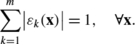Remark that the Bayes error rate of such a distribution corresponds to 1/m for the Hamming loss and 1−1/m for the subset 0/1 loss. Datasets following such a distribution can be easily generated, by sampling first without noise, and subsequently, by shifting at random one of the m labels in every label vector. One might expect that only substantial differences in performance will be observed for a small number of labels. Therefore, we only investigate the cases m=2,…,10.

In this case, the Bayes error rate for the Hamming loss decreases with the number of labels, while for subset 0/1 loss it increases. From the plots given in Fig. 6, we see that there is no single algorithm that can perform optimally for both loss functions simultaneously. From the bottom plot we can see that classifiers create a Pareto front, meaning that a trade-off can be observed between optimizing different loss functions from a multi-objective optimization perspective.

SBR and BR perform the best for the Hamming loss, with the former yielding a slight advantage. For the subset 0/1 loss, LP now becomes the best, thereby supporting our theoretical claim that BR is estimating marginal modes, while LP is seeking the mode of the joint conditional distribution. Moreover, an interesting behavior of CC can be observed; for a small number of labels, it properly estimates the joint mode. However, its performance decreases with an increase in the number of labels. It follows that one has to use a proper base classifier to capture the conditional dependence. A linear classifier is too weak in this case. Moreover, CC employs a greedy approximation of the joint mode, which might also have a negative impact on the performance.

Using these synthetic data, we also try to investigate the behavior of RAKEL and ECC. To this end, we used RAKEL with 10 LPs operating on random subsets of labels of size k∈{2,…,6}. The results for the problem with 8 labels are presented in Fig. 7. One can see a nice Pareto front of the algorithms, suggesting that RAKEL realizes a kind of trade-off between Hamming and subset 0-1 loss minimization. This is plausible, since this algorithm essentially reduces to BR for the extreme case k=1 and to LP for k=m (with m the number of labels). In addition, Fig. 7 visualizes the behavior of ECC with the number of iterations set to 5, 10, 15, and 20. Here we used synthetic data with 5 labels. One cannot observe a trend as obvious as in LP, but it seems that increasing the number of iterations moves the predictions from the joint mode into marginal modes.

### XOR problem

In the literature, LP is often shown to outperform BR even in terms of Hamming loss. Given our results so far, this is somewhat surprising and calls for an explanation. We argue that results of that kind should be considered with caution, mainly because a meta learning technique (such as BR and LP) must always be considered in conjunction with the underlying base learner. In fact, differences in performance should not only be attributed to the meta but also to the base learner. In particular, since BR uses binary and LP multi-class classification, they are typically applied with different base learners, and hence are not directly comparable.

We illustrate this by means of an example in which we generated data as before, but using XOR instead of linear models. More specifically, we first generated a linear model, and then converted it to an XOR problem by combining it with the corresponding orthogonal linear model. Each label depends on the same two features, but the parameters were generated independently for each label with τ=1. For simplicity, we did not use any kind of error.

Obviously, using a linear base learner, BR or SBR is not able to solve this problem properly, whereas LP, using a multi-class extension of LR (based on a one-vs-one decomposition) yields a good performance, for both loss functions. However, this multi-class extension is no longer a truly linear classifier. Instead, several linear classifiers are wrapped in a decomposition and an aggregation procedure, yielding a more complex classifier that can produce non-linear decision boundaries. And indeed, giving BR access to a more complex base learner, like the rule ensemble MLRules, it is able to solve the problem as well; see results and the scatter plot of data in Fig. 8.

### Benchmark data

The second part of the experiment concerns four benchmark datasets: scene, yeast, medical and emotions.Footnote 9 We used the original training and test sets given by the data providers. Thanks to that the results can be easily compared to future and already published studies. Below we present short description of each dataset, and Table 2 summarizes the main properties of them.

Scene is a semantic scene classification dataset proposed by Boutell et al. (2004), in which a picture can be categorized into one or more classes. In this dataset, pictures can have the following classes: beach, sunset, foliage, field, mountain, and urban. Features of this dataset correspond to spatial color moments in the LUV space. Color as well as spatial information have been shown to be fairly effective in distinguishing between certain types of outdoor scenes: bright and warm colors at the top of a picture may correspond to a sunset, while those at the bottom may correspond to a desert rock.

From the biological field, we have chosen the yeast dataset (Elisseeff and Weston 2002), which is about predicting the functional classes of genes in the Yeast Saccharomyces Cerevisiae. Each gene is described by the concatenation of microarray expression data and a phylogenetic profile, and associated with a set of 14 functional classes. The dataset contains 2417 genes in total, and each gene is represented by a 103-dimensional feature vector.

The medical (Pestian et al. 2007) dataset has been used in Computational Medicine Centers 2007 Medical Natural Language Processing Challenge.Footnote 10 It is a medical-text dataset that includes a brief free-text summary of patient symptom history and their prognosis, labeled with insurance codes. Each instance is represented with a bag-of-words of the symptom history and is associated with a subset of 45 labels (i.e., possible prognoses).

The emotions data was created from a selection of songs from 233 musical albums (Trohidis et al. 2008). From each song, a sequence of 30 seconds after the initial 30 seconds was extracted. The resulting sound clips were stored and converted into wave files of 22050 Hz sampling rate, 16-bit per sample and mono. From each wave file, 72 features have been extracted, falling into two categories: rhythmic and timbre. Then, in the emotion labeling process, 6 main emotional clusters are retained corresponding to the Tellegen-Watson-Clark model of mood: amazed-surprised, happy-pleased, relaxing-clam, quiet-still, sad-lonely and angry-aggressive.

Figure 9 visualizes the performance of eight classifiers on these datasets. We used four reduction methods for MLC: BR, SBR, PCC and LP along with LR and MLRules. The performance is presented in the Hamming vs. subset 0/1 loss space. The results are also summarized in Tables 3 and 4.

In general, the results confirm our theoretical claims. In the case of the yeast and emotions datasets, we can observe a kind of Pareto front of the classifiers. This suggests a strong conditional dependence between labels, resulting in different risk minimizers for Hamming and subset 0/1 loss. In the case of the scene and medical datasets, it seems that both risk minimizers coincide. The best algorithms perform equally good for both losses.

Moreover, one can also observe for the scene dataset that LP with a linear base classifier outperforms linear BR in terms of Hamming loss, but the use of a non-linear classifier in BR improves the results again over LP. As pointed out above, comparing LP and BR with the same base learner is questionable and may lead to unwarranted conclusions. Similar to the synthetic XOR experiment, performance gains of LP and CC might be primarily due to a hypothesis space extension, especially because the methods with nonlinear base learners perform well in general.

## Conclusions

In this paper, we have addressed a number of issues around one of the core topics in current MLC research, namely the idea of improving predictive performance by exploiting label dependence. In our opinion, this topic has not received enough attention so far, despite the increasing interest in MLC in general. Indeed, as we have argued in this paper, empirical studies of MLC methods are often meaningless or even misleading without a careful interpretation, which in turn requires a thorough understanding of underlying theoretical conceptions.

In particular, by looking at the current literature, we noticed that papers proposing new methods for MLC rarely give a precise definition of the type of dependence they have in mind, despite stating the exploitation of label dependence as an explicit goal. Besides, the type of loss function to be minimized, i.e., the concrete goal of the classifier, is often not mentioned either. Instead, a new method is shown to be better than existing ones “on average”, evaluating on a number of different loss functions.

Based on a distinction between two types of label dependence that seem to be important in MLC, namely marginal and conditional dependence, we have established a close connection between the type of dependence present in the data and the type of loss function to be minimized. In this regard, we have also distinguished three classes of problem tasks in MLC, namely the minimization of single-label loss functions, multi-label loss functions, and the estimation of the joint conditional distribution.

On the basis of our theoretical results, in conjunction with several empirical studies using both synthetic and benchmark data, we can draw a couple of conclusions:

• The type of loss function has a strong influence on whether or not, and perhaps to what extent, an exploitation of label dependencies can be expected to yield a true benefit.

• Marginal label dependence can help in boosting the performance for single-label and multi-label loss functions that have marginal conditional distributions as risk minimizers, while conditional dependence plays a role for loss functions having a more complex risk minimizer, such as the subset 0/1 loss, which requires estimating the mode of the joint conditional distribution.

• Loss functions in MLC are quite diverse, and minimizing different losses will normally require different estimators. Using the Hamming and subset 0/1 loss as concrete examples, we have shown that a minimization of the former may cause a high regret for the latter and vice versa.

We believe that these results have a number of important implications, not only from a theoretical but also from a methodological and practical point of view. Perhaps most importantly, one cannot expect the same MLC method to be optimal for different types of losses at the same time, and each new approach shown to outperform others across a wide and diverse spectrum of different loss functions should be considered with reservation. Besides, more efforts should be made in explaining the improvements that are achieved by an algorithm, laying bare its underlying mechanisms, the type of label dependence it assumes, and the way in which this dependence is exploited. Since experimental studies often contain a number of side effects, relying on empirical results alone, without a careful analysis and reasonable explanation, appears to be disputable.

1. For a predicate P, the expression [[P]] evaluates to 1 if P is true and to 0 if P is false.

2. Please note that we use the term “marginal distribution” with two different meanings, namely for P(Y) (marginalization over the joint distribution P(X,Y)) and for P(Y i |x) (marginalization over P(Y|x)).

3. Note that the denominator is 0 if y i =h i (x)=0 for all i=1,…,m. In this case, the loss is 0 by definition. The same remark applies to the Jaccard distance.

4. Methods of type (26) can also be used in order to reduce computational costs. By transforming the output space to a new space of lower dimension, we end up with solving a fewer number of core problems.

5. All the datasets have been taken from the MULAN repository http://mulan.sourceforge.net.

6. We will suppress dependence on x in the notation, whenever it is clear from the context.

## References

• Amit, Y., Dekel, O., & Singer, Y. (2007). A boosting algorithm for label covering in multilabel problems. In JMLR W&P (Vol. 2, pp. 27–34).

• an der Merwe, A., & Zidek, J. (1980). Multivariate regression analysis and canonical variates. Canadian Journal of Statistics, 8, 27–39.

• Boutell, M., Luo, J., Shen, X., & Brown, C. (2004). Learning multi-label scene classification. Pattern Recognition, 37(9), 1757–1771.

• Breiman, L., & Friedman, J. (1997). Predicting multivariate responses in multiple linear regression. Journal of the Royal Statistical Society. Series B. Statistical Methodology, 69, 3–54.

• Caruana, R. (1997). Multitask learning: A knowledge-based source of inductive bias. Machine Learning, 28, 41–75.

• Cheng, W., & Hüllermeier, E. (2009). Combining instance-based learning and logistic regression for multilabel classification. Machine Learning, 76(2-3), 211–225.

• Chierichetti, F., Kumar, R., Pandey, S., & Vassilvitskii, S. (2010). Finding the Jaccard median. In ACM-SIAM SODA 2010 (pp. 293–311).

• Dekel, O., Manning, C., & Singer, Y. (2004). Log-linear models for label ranking. In S. Thrun, L. Saul, & B. Schölkopf (Eds.), NIPS 16. Cambridge: MIT Press.

• Dembczyński, K., Kotłowski, W., & Słowiński, R. (2008). Maximum likelihood rule ensembles. In ICML 2008 (pp. 224–231). Madison: Omnipress.

• Dembczyński, K., Cheng, W., & Hüllermeier, E. (2010a). Bayes optimal multilabel classification via probabilistic classifier chains. In ICML 2010. Madison: Omnipress.

• Dembczyński, K., Waegeman, W., Cheng, W., & Hüllermeier, E. (2010b). On label dependence in multi-label classification. In Second international workshop on learning from multi-label data (MLD 2010), in conjunction with ICML/COLT 2010.

• Dembczyński, K., Waegeman, W., Cheng, W., & Hüllermeier, E. (2010c). Regret analysis for performance metrics in multi-label classification: The case of hamming and subset zero-one loss. In ECML/PKDD 2010. Berlin: Springer.

• Dembczyński, K., Waegeman, W., Cheng, W., & Hüllermeier, E. (2012) An exact algorithm for F-measure maximization. In Advances in neural information processing systems (Vol. 25).

• Elisseeff, A., & Weston, J. (2002). A kernel method for multi-labelled classification. In NIPS 14 (pp. 681–688).

• Finley, T., & Joachims, T. (2008). Training structural SVMs when exact inference is intractable. In ICML 2008. Madison: Omnipress.

• Fürnkranz, J., Hüllermeier, E., Loza Mencía, E., & Brinker, K. (2008). Multilabel classification via calibrated label ranking. Machine Learning, 73(2).

• Ghamrawi, N., & McCallum, A. (2005). Collective multi-label classification. In CIKM 2005 (pp. 195–200).

• Godbole, S., & Sarawagi, S. (2004). Discriminative methods for multi-labeled classification. In PAKDD 2004 (pp. 22–30).

• Hariharan, B., Zelnik-Manor, L., Vishwanathan, S., & Varma, M. (2010). Large scale max-margin multi-label classification with priors. In ICML 2010. Berlin: Omnipress.

• Hastie, T., Tibshirani, R., & Friedman, J. H. (2007). Elements of statistical learning: data mining, inference, and prediction (2nd ed.). Berlin: Springer.

• Hsu, D., Kakade, S., Langford, J., & Zhang, T. (2009). Multi-label prediction via compressed sensing. In NIPS 22 (pp. 772–780).

• Hüllermeier, E., Fürnkranz, J., Cheng, W., & Brinker, K. (2008). Label ranking by learning pairwise preferences. Artificial Intelligence, 172(16–17), 1897–1916.

• Izenman, A. (1975). Reduced-rank regression for the multivariate linear model. Journal of Multivariate Analysis, 5, 248–262.

• Joe, H. (2000). Multivariate models and dependence concepts. London: Chapman & Hall.

• Jordan, M. I. (Ed.) (1998). Learning in graphical models. Dordrecht: Kluwer Academic.

• Karush, W. (1939). Minima of functions of several variables with inequalities as side constraints. Master’s thesis, Dept. of Mathematics, Univ. of Chicago.

• Kuhn, H. W., & Tucker, A. W. (1951). Nonlinear programming. In Proceedings of the second Berkeley symposium on mathematical statistics and probability (pp. 481–492).

• Lafferty, J. D., McCallum, A., & Pereira, F. C. N. (2001). Conditional random fields: Probabilistic models for segmenting and labeling sequence data. In ICML 2001 (pp. 282–289).

• Pestian, J. P., Brew, C., Matykiewicz, P., Hovermale, D. J., Johnson, N., Cohen, K. B., & Duch, W. (2007). A shared task involving multi-label classification of clinical free text. In BioNLP’07: proceedings of the workshop on BioNLP 2007 (pp. 97–104). Association for Computational Linguistics.

• Pletscher, P., Ong, C. S., & Buhmann, J. M. (2010). Entropy and margin maximization for structured output learning. In ECML/PKDD 2010. Berlin: Springer.

• Read, J., Pfahringer, B., Holmes, G., & Frank, E. (2009). Classifier chains for multi-label classification. In ECML/PKDD 2009 (pp. 254–269).

• Schapire, RE, & Singer, Y. (2000). Boostexter: A boosting-based system for text categorization. Machine Learning, 39, 135–168.

• Sklar, A. (1959). Functions de répartitions à n dimensions et leurs marges (Tech. rep.). Public Institute of Statistics of the University of Paris 8.

• Tai, F., & Lin, H. T. (2010). Multi-label classification with principle label space transformation. In: Second international workshop on learning from multi-label data, (MLD 2010), in conjunction with ICML/COLT 2010.

• Trohidis, K., Tsoumakas, G., Kalliris, G., & Vlahavas, I. (2008). Multi-label classification of music into emotions. In ISMIR 2008 (pp. 325–330).

• Tsochantaridis, Y., Joachims, T., Hofmann, T., & Altun, Y. (2005). Large margin methods for structured and independent output variables. Journal of Machine Learning Research, 6, 1453–1484.

• Tsoumakas, G., & Katakis, I. (2007). Multi label classification: An overview. International Journal of Data Warehousing and Mining, 3(3), 1–13.

• Tsoumakas, G., & Vlahavas, I. (2007). Random k-labelsets: An ensemble method for multilabel classification. In ECML 2007 (pp. 406–417).

• Tsoumakas, G., Katakis, I., & Vlahavas, I. (2010). Mining multi-label data. In O. Maimon & L. Rokach (Eds.), Data mining and knowledge discovery handbook. Berlin: Springer.

• Weston, J., Chapelle, O., Elisseeff, A., Schölkopf, B., & Vapnik, V. (2002). Kernel dependency estimation. In NIPS 2002 (pp. 873–880).

• Witten, I. H., & Frank, E. (2005). Data mining: practical machine learning tools and techniques (2nd ed.). San Mateo: Morgan Kaufmann.

• Yu, S., Yu, K., Tresp, V, & Kriegel, H. P. (2006). Multi-output regularized feature projection. IEEE Transactions on Knowledge and Data Engineering, 18(12), 1600–1613.

• Zhang, M. L., & Zhang, K. (2010). Multi-label learning by exploiting label dependency. In Proceedings of the 16th ACM SIGKDD international conference on knowledge discovery and data mining (pp. 999–1008). New York: ACM.

## Acknowledgements

Krzysztof Dembczyński has started this work during his post-doctoral stay at Marburg University supported by the German Research Foundation (DFG) and finalized it at Poznań University of Technology under the grant 91-515/DS of the Polish Ministry of Science and Higher Education. Willem Waegeman is supported as a postdoc by the Research Foundation of Flanders (FWO-Vlaanderen). The part of this work has been done during his visit at Marburg University. Weiwei Cheng and Eyke Hüllermeier are supported by DFG. The authors are grateful to the anonymous reviewers for their valuable comments and suggestions.

## Author information

Authors

### Corresponding author

Correspondence to Krzysztof Dembczyński.

Editors: Grigorios Tsoumakas, Min-Ling Zhang, and Zhi-Hua Zhou

## Appendix

### Theorem 1

A ranking function that sorts the labels according to their probability of relevance, i.e., using the scoring function h(⋅) with

$$h_i(\mathbf{x}) = \mathbf {P}(Y_i = 1 | \mathbf{x}),$$
(28)

minimizes the expected rank loss (17).

### Proof

The risk of a scoring vector f=f(x) can be written as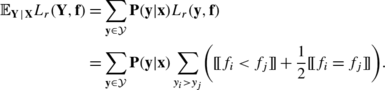The two sums can be swapped, and doing so yields the expression

$$\sum\limits _{1 \le i , j \le m} \sum\limits _{\mathbf{y} \in\mathcal{Y}} \mathbf {P}(\mathbf{y} | \mathbf{x})[\![y_i>y_j ]\!]\biggl([\![f_i<f_j ]\!]+ \frac{1}{2} [\![f_i=f_j ]\!]\biggr)$$

which in turn can be written as

$$\sum\limits _{1 \le i < j \le m} g(i,j) + g(j,i)$$

with

$$g(i,j) = \mathbf {P}(y_i > y_j | \mathbf{x}) \biggl([\![f_i < f_j ]\!]+ \frac{1}{2} [\![f_i=f_j ]\!]\biggr).$$

For each pair of labels y i ,y j , the sum g(i,j)+g(j,i) is obviously minimized by choosing the scores f i ,f j such that f i <f j whenever P(y i >y j |x)<P(y j >y i |x), f i =f j whenever P(y i >y j |x)=P(y j >y i |x), and f i >f j whenever P(y i >y j |x)>P(y j >y i |x). Since

$$\mathbf {P}(y_i>y_j|\mathbf{x}) - \mathbf {P}(y_j > y_i | \mathbf{x}) = \mathbf {P}(y_i = 1|\mathbf{x}) - \mathbf {P}(y_j = 1 | \mathbf{x}),$$

the minimizer can be expressed in terms of P(y i =1|x) and P(y j =1|x). Consequently, the scores (28) minimize the sums g(i,j)+g(j,i) simultaneously for all label pairs and, therefore, minimize risk. □

### Proposition 3

For all distributions of Y given x, and for all models h, the expectation of the subset 0/1 loss can be bounded in terms of the expectation of the Hamming loss as follows:### Proof

For a fixed $$\mathbf{x} \in\mathcal{X}$$, we can express the expected loss as follows: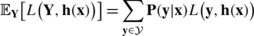Suppose we can express an MLC loss in terms of an aggregation G:{0,1}m→[0,1] of the standard zero-one losses L 0/1 on individual labels (as used in conventional classification):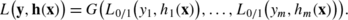Indeed, the subset 0/1 loss and the Hamming loss can be written, respectively, as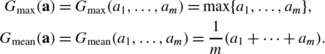This immediately leads to the above lower and upper bound for the Hamming loss. The proposition then immediately follows from the fact that $$\frac{1}{m} G_{\mathrm{max}}(\mathbf{a}) \leq G_{\mathrm{mean}}(\mathbf {a}) \leq G_{\mathrm{max}}(\mathbf{a})$$ for all a∈[0,1]m. □

### Proposition 4

The following upper bound holds:

$$\mathbb{E}_{\mathbf{Y}} L_s\bigl(\mathbf{Y}, \mathbf{h}^*_H(\mathbf{x})\bigr) - \mathbb{E}_{\mathbf{Y}} L_s\bigl(\mathbf{Y}, \mathbf{h}^*_s(\mathbf{x})\bigr) <0.5$$

Moreover, this bound is tight, i.e.,

$$\sup_{\mathbf {P}} \bigl(\mathbb{E}_{\mathbf{Y}} L_s\bigl( \mathbf{Y}, \mathbf {h}^*_H(\mathbf{x})\bigr) - \mathbb{E}_{\mathbf{Y}} L_s\bigl(\mathbf{Y}, \mathbf {h}^*_s(\mathbf{x})\bigr) \bigr) = 0.5,$$

where the supremum is taken over all probability distributions on $$\mathcal{Y}$$.

### Proof

Since the risk of any classifier h is within the range [0,1], the maximal value of the regret is 1. However, according to the second part of Proposition 2, both risk minimizers coincide if $$\mathbb{E}_{\mathbf{Y}} L_{s}(\mathbf {Y}, \mathbf{h}^{*}_{s}(\mathbf{x})) \le0.5$$. Consequently, the regret must be (strictly) smaller than 0.5. To prove the tightness of the bound, we show that, for any $$\delta\in(0,\frac{1}{6})$$, there is a probability distribution P that yields the regret 0.5−3δ. Define P as follows: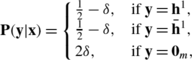where h 1 represents an m-dimensional vector of zeros, apart from a one at the first position, and $$\mathbf{\bar{h}}^{1}$$ corresponds to the negation of h 1. Such a distribution can be constructed for all m>1. Obviously, then $$\mathbf{h}^{*}_{s}(\mathbf{x})$$ corresponds to h 1 or $$\mathbf{\bar {h}}^{1}$$ and $$\mathbf{h}^{*}_{H}(\mathbf{x})$$ becomes 0 m . Finally, we thus obtain

$$\mathbb{E}_{\mathbf{Y}} L_s\bigl(\mathbf{Y}, \mathbf{h}^*_H(\mathbf{x})\bigr) = 1 - 2\delta$$

and

$$\mathbb{E}_{\mathbf{Y}} L_s\bigl(\mathbf{Y}, \mathbf{h}^*_s(\mathbf{x})\bigr) = 0.5 + \delta,$$

which immediately proves the proposition. □

### Proposition 5

The following upper bound holds for m>3:

$$\mathbb{E}_{\mathbf{Y}} L_H\bigl(\mathbf{Y}, \mathbf{h}^*_s(\mathbf{x})\bigr) - \mathbb{E}_{\mathbf{Y}} L_H\bigl(\mathbf{Y}, \mathbf{h}^*_H(\mathbf{x})\bigr) < \frac{m-2}{m+2}.$$

Moreover, this bound is tight, i.e.

$$\sup_{\mathbf {P}}\bigl(\mathbb{E}_{\mathbf{Y}} L_H\bigl( \mathbf{Y}, \mathbf{h}^*_s(\mathbf {x})\bigr) - \mathbb{E}_{\mathbf{Y}} L_H\bigl(\mathbf{Y}, \mathbf{h}^*_H(\mathbf{x})\bigr) \bigr) = \frac{m-2}{m+2},$$

where the supremum is taken over all probability distributions on $$\mathcal{Y}$$.

### Proof

We first show that there is a distribution yielding regret arbitrarily close to the bound, before proving the tightness. Let a i ∈{0,1} and $$\bar{a}_{i} = 1 - a_{i}$$. If a m =(a 1,a 2,…,a m ) is a {0,1}-vector of length m, then $$\mathbf{\bar{a}}_{m}$$ denotes the vector $$(\bar{a}_{1}, \bar{a}_{2}, \ldots, \bar{a}_{m})$$. Furthermore, let d H (a,b) denote the Hamming distance, given by

$$d_H(\mathbf{a},\mathbf{b}) = \frac{1}{m}\sum _{i=1}^m |a_i - b_i|$$

for all a,b∈{0,1}m. Now, consider a joint probability distribution defined as follows:Footnote 11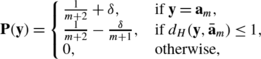where δ>0. Hence, we obtain $$\mathbf{h}^{*}_{H} = \bar{a}_{m}$$ for small enough δ and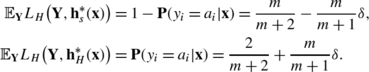The difference is then given by

$$\mathbb{E}_{\mathbf{Y}} L_H\bigl(\mathbf{Y}, \mathbf{h}^*_s(\mathbf{x})\bigr) - \mathbb{E}_{\mathbf{Y}} L_H\bigl(\mathbf{Y}, \mathbf{h}^*_H(\mathbf{x})\bigr) = \frac{m-2}{m+2} - \delta\frac{2m}{m+1}.$$

Since this holds for any δ>0, the regret gets arbitrarily close to the bound.

Additionally, we show that the right-hand side of the inequality in the proposition is an upper bound, which is more involved. To this end, we will show that maximizing the regret over all probability distributions can be bounded by several linear programs, with optimal values bounded by the right-hand side. Let us introduce

$$\Delta L_m^{\max} = \sup_{\mathbf {P}} \Delta L_m (\mathbf {P}) = \sup_{\mathbf {P}} \bigl(\mathbb {E}_{\mathbf{Y}} L_H\bigl(\mathbf{Y}, \mathbf{h}^*_s(\mathbf{x})\bigr) - \mathbb {E}_{\mathbf{Y}} L_H\bigl(\mathbf{Y}, \mathbf{h}^*_H( \mathbf{x})\bigr)\bigr).$$

Let us first consider an arbitrary probability distribution. Without loss of generality, we can permute labels in such a way that the zero vector 0 m , containing m zeros, corresponds to the mode. The Hamming loss of the subset 0/1 loss minimizer and Hamming loss minimizer can then be expressed as: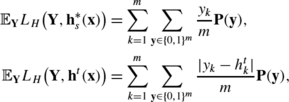where y i denotes the ith entry of the vector y and h t represents any multi-label classifier consisting of t ones and mt zeros. Again without loss of generality, we can further permute indices in such a way that the Hamming loss minimizer is given by first t ones, followed by mt zeros, where 1≤tm. As a consequence, we find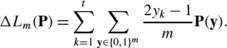Furthermore, $$\Delta L_{m}^{\max}$$ is upper bounded by the solution of the following optimization problem: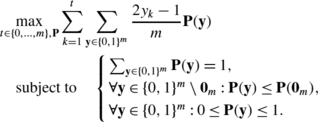(29)

This is a mixed integer linear program with t the only integer variable. Its solution only acts as an upper bound since we introduced two relaxations: the subset zero-one loss minimizer can now coincide with the Hamming loss minimizer and it consists of t ones followed by mt zeros. For t fixed, the optimization problem becomes a regular linear program. As a consequence, let us first solve for P(y) and subsequently for t: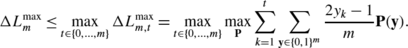We show that for a given t the solution $$\Delta L_{m,t}^{\max}$$ of the linear program is given by P A when t≥2mt+1 and by P B when t≤2mt+1. These distributions are defined as follows: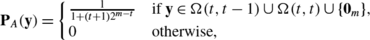orwhere the set Ω(p,q) is defined as:

$$\Omega(p,q) = \Biggl\{\mathbf{y} \in\{0,1\}^m \mid\sum _{i=1}^p y_i = q\Biggr\}.$$

For these probability distributions, the value of the objective function is respectively given by: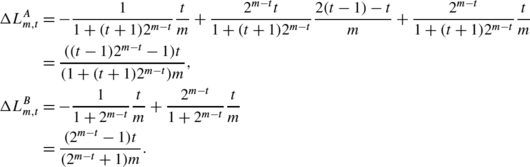To show that one of these two probability distributions defines the maximum of the linear program, we verify the Karush-Kuhn-Tucker conditions (Karush 1939; Kuhn and Tucker 1951) for a given value of t. If t is fixed, (29) can be simplified to the following standard linear program form: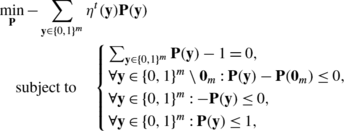with

$$\eta^t(\mathbf{y}) = \sum_{k=1}^t y_k.$$

The primal Lagrangian can be defined as: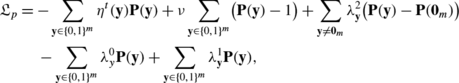with $$\nu, \lambda^{0}_{\mathbf{y}}, \lambda^{1}_{\mathbf{y}}$$ and $$\lambda^{2}_{\mathbf{y}}$$ Lagrange multipliers. The stationarity condition for optimality implies that the gradient of the primal Lagrangian equals zero, leading to the following system of linear equations: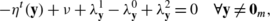(30)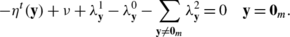(31)

Other conditions that need to be satisfied are dual feasibility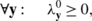(32)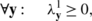(33)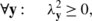(34)

and the complementary slackness conditions, which are different for P A (y) and P B (y). For P A (y) they are given by: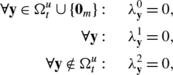where $$\Omega^{u}_{t} = \Omega(t,t) \cup\Omega(t,t-1)$$. Plugging the latter three conditions into (30) and (31) yields

$$\begin{array}{l@{\quad}l} \lambda^2_{\mathbf{y}} = t - v, & \forall\mathbf{y} \in\Omega(t,t),\\[2pt] \lambda^2_{\mathbf{y}} = t - 1 - v, & \forall\mathbf{y} \in\Omega(t,t-1),\\[2pt] \lambda^0_{\mathbf{y}} = - \eta^t(\mathbf{y}) + v, &\forall\mathbf{y}\notin\Omega^u_t \cup\{\mathbf{0}_m\},\\ [2pt] v = 2^{m-t}(t-v) + t 2^{m-t} (t-1-v). & \end{array}$$

Solving the last equation for v results in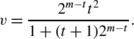Finally, we only need to verify the dual feasibility conditions: (32) and (33) are always satisfied, (34) is satisfied as soon as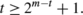(35)

So, P A (y) delivers the optimum for all t and m that satisfy (35). In a very similar way, one can show that P B (y) becomes optimal when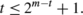(36)

As a result, either P A (y) or P B (y) can be the optimum for a given t and m. They are both solutions to the optimization problem for the specific case where the above inequality becomes an equality. Remark that also other solutions exist only for this specific case.

We observe that P A (y) yields for t=m the regret mentioned in the proposition. Hence, we only need to show that this fraction is indeed the maximum value of the objective function for all values of t, if P A (y) and P B (y) are considered. Both $$\Delta L_{m,t}^{A}$$ and $$\Delta L_{m,t}^{B}$$ are upper bounded by t/m, which is further upper bounded by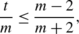for all t∈{1,…,m−4}. We complete the proof by verifying manually the difference in the objective function value for the remaining values of t. This leads to the following bound that needs to be satisfied for P A (y):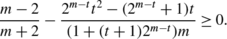As a result, one obtains for t∈{m−3,m−2,m−1} respectively: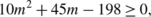(37)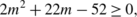(38)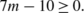(39)

The following bound needs to be satisfied for P B (y):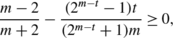resulting in the following inequalities for t∈{m−3,m−2,m−1,m} respectively: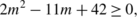(40)(41)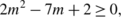(42)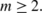(43)

It turns out that all inequalities (37)–(43) are simultaneously satisfied for m>3. □

## Rights and permissions

Reprints and Permissions

Dembczyński, K., Waegeman, W., Cheng, W. et al. On label dependence and loss minimization in multi-label classification. Mach Learn 88, 5–45 (2012). https://doi.org/10.1007/s10994-012-5285-8

• Accepted:

• Published:

• Issue Date:

• DOI: https://doi.org/10.1007/s10994-012-5285-8

### Keywords

• Multi-label classification
• Label dependence
• Loss functions